##### The Japanese Maple specialist
Direct order Contact Help / Services Newsletter# Shelf to displaySearch

Japanese MaplesYoung trees Acer seeds and more Bonsai soil and fertiliserVarieties introduced into EuropeHostasWind bells and chimesSaxifragesSpecial offer

Packing and shipping charges

# Tamahi small size organic bonsai fertilizer

Tamahi engrais bonsai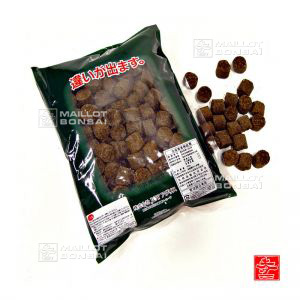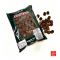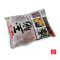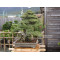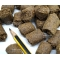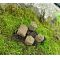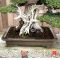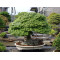ref. : 6807

34,50

Available quantity : 7Order

###### Description

Organic manure in 1 cm diameter pellets. Bag 3 kilograms.

For use especially on outdoor bonsai. N-P-K: 5.4.1. The most popular fertiliser product used by Japanese gardening professionals.

How to use: put the pellets on the earth at the beginning of spring, summer and autumn.

Dosage: for a 20 cm pot use 3 to 4 grains every 45 days. This dose can be adjusted depending on how fast you want your plants and trees to grow.

#fertilizer 5 #organic 3.7 #bonsai 3.6 #pellets 2.7 #tamahi 2.6 #small 2.5 #size 2.4 #professionals 2.3 #especially 2 #fertiliser 2

Formule
(( ROUND((CHAR_LENGTH(b.article_nom)-CHAR_LENGTH(REPLACE(b.article_nom, 'organic', '')))/LENGTH('organic')) + ROUND((CHAR_LENGTH(b.article_description)-CHAR_LENGTH(REPLACE(b.article_description, 'organic', '')))/LENGTH('organic')) ) * 3.7) + (( ROUND((CHAR_LENGTH(b.article_nom)-CHAR_LENGTH(REPLACE(b.article_nom, 'bonsai', '')))/LENGTH('bonsai')) + ROUND((CHAR_LENGTH(b.article_description)-CHAR_LENGTH(REPLACE(b.article_description, 'bonsai', '')))/LENGTH('bonsai')) ) * 3.6) + (( ROUND((CHAR_LENGTH(b.article_nom)-CHAR_LENGTH(REPLACE(b.article_nom, 'fertilizer', '')))/LENGTH('fertilizer')) + ROUND((CHAR_LENGTH(b.article_description)-CHAR_LENGTH(REPLACE(b.article_description, 'fertilizer', '')))/LENGTH('fertilizer')) ) * 3) + (( ROUND((CHAR_LENGTH(b.article_nom)-CHAR_LENGTH(REPLACE(b.article_nom, 'pellets', '')))/LENGTH('pellets')) + ROUND((CHAR_LENGTH(b.article_description)-CHAR_LENGTH(REPLACE(b.article_description, 'pellets', '')))/LENGTH('pellets')) ) * 2.7) + (( ROUND((CHAR_LENGTH(b.article_nom)-CHAR_LENGTH(REPLACE(b.article_nom, 'tamahi', '')))/LENGTH('tamahi')) + ROUND((CHAR_LENGTH(b.article_description)-CHAR_LENGTH(REPLACE(b.article_description, 'tamahi', '')))/LENGTH('tamahi')) ) * 2.6) + (( ROUND((CHAR_LENGTH(b.article_nom)-CHAR_LENGTH(REPLACE(b.article_nom, 'small', '')))/LENGTH('small')) + ROUND((CHAR_LENGTH(b.article_description)-CHAR_LENGTH(REPLACE(b.article_description, 'small', '')))/LENGTH('small')) ) * 2.5) + (( ROUND((CHAR_LENGTH(b.article_nom)-CHAR_LENGTH(REPLACE(b.article_nom, 'size', '')))/LENGTH('size')) + ROUND((CHAR_LENGTH(b.article_description)-CHAR_LENGTH(REPLACE(b.article_description, 'size', '')))/LENGTH('size')) ) * 2.4) + (( ROUND((CHAR_LENGTH(b.article_nom)-CHAR_LENGTH(REPLACE(b.article_nom, 'professionals', '')))/LENGTH('professionals')) + ROUND((CHAR_LENGTH(b.article_description)-CHAR_LENGTH(REPLACE(b.article_description, 'professionals', '')))/LENGTH('professionals')) ) * 2.3) + (( ROUND((CHAR_LENGTH(b.article_nom)-CHAR_LENGTH(REPLACE(b.article_nom, 'especially', '')))/LENGTH('especially')) + ROUND((CHAR_LENGTH(b.article_description)-CHAR_LENGTH(REPLACE(b.article_description, 'especially', '')))/LENGTH('especially')) ) * 2) + (( ROUND((CHAR_LENGTH(b.article_nom)-CHAR_LENGTH(REPLACE(b.article_nom, 'fertiliser', '')))/LENGTH('fertiliser')) + ROUND((CHAR_LENGTH(b.article_description)-CHAR_LENGTH(REPLACE(b.article_description, 'fertiliser', '')))/LENGTH('fertiliser')) ) * 2)

## Secure payment## Delivery

Our logistic partners :04 74 55 23 48
Pépinière MAILLOT-BONSAÏ
Le Bois Frazy
01990 RELEVANT - FRANCE
on appointment